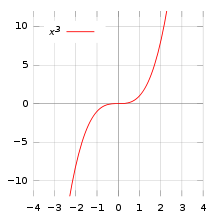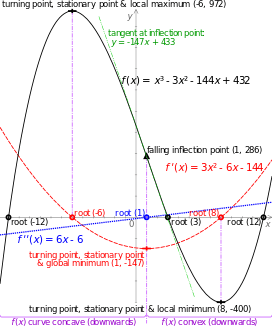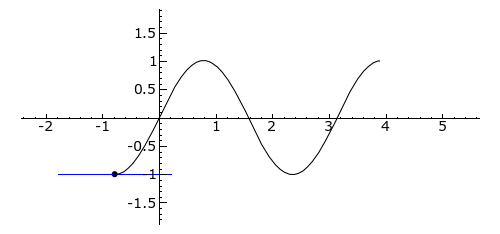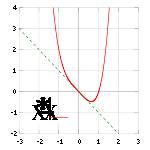# Inflection pointPlot of y = x³ with inflection point of (0,0), also a saddle point.The roots, turning points, stationary points, inflection point and concavity of a cubic polynomial x3 − 3x2 − 144x + 432 (black line) and its first and second derivatives (red and blue).

In differential calculus, an inflection point, point of inflection, flex, or inflection (inflexion (British English)) is a point on a curve at which the curve changes from being concave (concave downward) to convex (concave upward), or vice versa.

A point where the curvature vanishes but does not change sign is sometimes called a point of undulation or undulation point.

In algebraic geometry an inflection point is defined slightly more generally, as a point where the tangent meets the curve to order at least 3, and an undulation point or hyperflex is defined as a point where the tangent meets the curve to order at least 4.

## Definition

Inflection points are the points of the curve where the curvature changes its sign while a tangent exists.

A differentiable function has an inflection point at (x, f(x)) if and only if its first derivative, f′, has an isolated extremum at x. (This is not the same as saying that f has an extremum). That is, in some neighborhood, x is the one and only point at which f′ has a (local) minimum or maximum. If all extrema of f′ are isolated, then an inflection point is a point on the graph of f at which the tangent crosses the curve.

A rising point of inflection is an inflection point where the derivative has a local minimum, and a falling point of inflection is a point where the derivative has a local maximum.

For an algebraic curve, a non singular point is an inflection point if and only if the multiplicity of the intersection of the tangent line and the curve (at the point of tangency) is odd and greater than 2.

For a curve given by parametric equations, a point is an inflection point if its signed curvature changes from plus to minus or from minus to plus, i.e., changes sign.

For a twice differentiable function, an inflection point is a point on the graph at which the second derivative has an isolated zero and changes sign.Plot of f(x) = sin(2x) from −π/4 to 5π/4; note f’s second derivative is f″(x) = –4sin(2x). Tangent is blue where the curve is convex (above its own tangent), green where concave (below its tangent), and red at inflection points: 0, π/2 and π

## A necessary but not sufficient condition

If x is an inflection point for f then the second derivative, f″(x), is equal to zero if it exists, but this condition does not provide a sufficient definition of a point of inflection. One also needs the lowest-order (above the second) non-zero derivative to be of odd order (third, fifth, etc.). If the lowest-order non-zero derivative is of even order, the point is not a point of inflection, but an undulation point. However, in algebraic geometry, both inflection points and undulation points are usually called inflection points. An example of such an undulation point is x = 0 for the function f given by f(x) = x4.

This definition assumes that f has some higher-order non-zero derivative at x, which is not necessarily the case, But if it has one, it follows from the definition that the sign of f′(x) is the same on either side of x in a neighborhood of x. If this is positive, the point is a rising point of inflection; if it is negative, the point is a falling point of inflection.

Inflection points sufficient conditions:

1) A sufficient existence condition for a point of inflection is:

If f(x) is k times continuously differentiable in a certain neighbourhood of a point x with k odd and k ≥ 3, while f(n)(x0)=0 for n = 2,...,k - 1 and f(k)(x0) ≠ 0 then f(x) has a point of inflection at x0.

2) Another sufficient existence condition requires f′′(x + ε) and f′′(x - ε) to have opposite signs in the neighborhood of x , if also a tangent exists here. (Bronshtein and Semendyayev 2004, p. 231).

## Categorization of points of inflection

Points of inflection can also be categorized according to whether f′(x) is zero or not zero.

• if f′(x) is zero, the point is a stationary point of inflection
• if f′(x) is not zero, the point is a non-stationary point of inflectiony = x4x has a 2nd derivative of zero at point (0,0), but it is not an inflection point because the fourth derivative is the first higher order non-zero derivative (the third derivative is zero as well).

An example of a saddle point is the point (0,0) on the graph y = x3. The tangent is the x-axis, which cuts the graph at this point.

A non-stationary point of inflection can be visualised if the graph y = x3 is rotated slightly about the origin. The tangent at the origin still cuts the graph in two, but its gradient is non-zero.

## Functions with discontinuities

Some functions change concavity without having points of inflection. Instead, they can change concavity around vertical asymptotes or discontinuities. Take, for example, the function 2x2/(x2 – 1). It is concave when |x| > 1 and convex when |x| < 1. However, it has no points of inflection because 1 and -1 are not in the domain of the function.